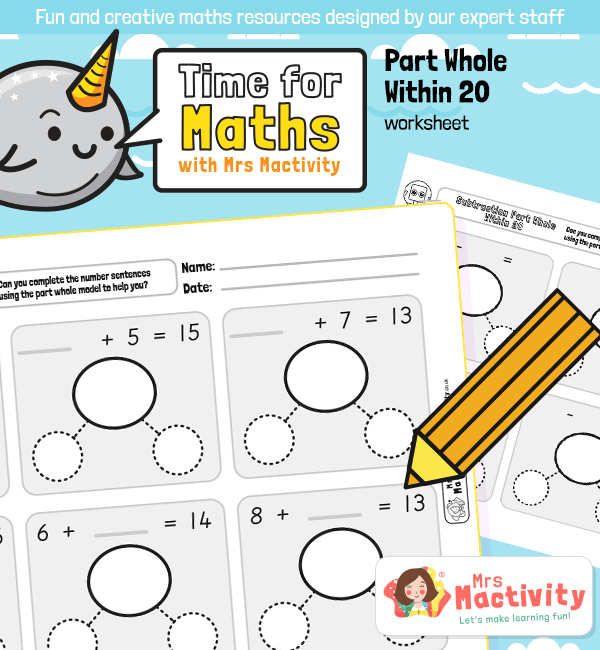Related Number Facts – Part Whole Addition and Subtraction Within 20 WorksheetsHelp children develop maths mastery skills with our Related Number Facts – Part Whole Addition and Subtraction Within 20 Worksheets. Helping children to understand how addition and subtraction relate to one another is a great way to help children acquire maths knowledge, skills and understanding, and is brilliant for building up maths confidence. Using the CPA (concrete-pictorial-abstract) approach is a really effective approach to maths that develops a deep and sustainable understanding, using physical and visual aids as a way to deepen children’s understanding.

National Curriculum Objectives

• Represent and use number bonds and related subtraction facts within 20
• Add and subtract one-digit and two-digit numbers to 20, including zero
• Read, write and interpret mathematical statements involving addition (+), subtraction (–) and equals (=) signs

Aligned with the White Rose Maths teaching sequence, this maths lesson presentation could be taught in Spring block 1 (addition and subtraction – related facts).

Explore our year 1 maths resources.

Explore our maths scheme.

Explore our addition and subtraction resources.